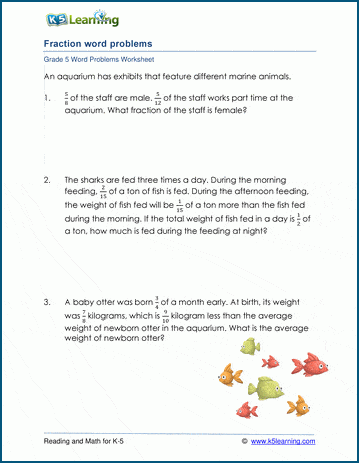# Add & subtract fractions word problems

## Word problem worksheets: Add & subtract fractions

Below are three versions of our grade 5 math word problem worksheet on adding and subtracting fractions.  The solutions to the problems will required addition or subtraction of fractions with both like and unlike denominators, and may include more than two terms.These worksheets are pdf files.## More word problem worksheets

Explore all of our math word problem worksheets, from kindergarten through grade 5.

## What is K5?

K5 Learning offers reading and math worksheets, workbooks and an online reading and math program for kids in kindergarten to grade 5.  We help your children build good study habits and excel in school.